Computational & Technology Resources
an online resource for computational,
engineering & technology publications
Civil-Comp Proceedings
ISSN 1759-3433
CCP: 84
PROCEEDINGS OF THE FIFTH INTERNATIONAL CONFERENCE ON ENGINEERING COMPUTATIONAL TECHNOLOGY
Edited by: B.H.V. Topping, G. Montero and R. Montenegro
Paper 21

Computer Simulations for a Fractional Calculus Derived Internet Traffic Model

C. Aguirre1, D. Campos1, P. Pascual1 and L. Vázquez23

1GNB, Department of Engineering Informatics, Escuela Politécnica Superior, Universidad Autónoma of Madrid, Spain
2Department of Applied Mathematics, Faculty of Informatics, Universidad Complutense of Madrid, Spain
3Centre for Astrobiology (CSIC-INTA), Torrejón de Ardoz, Madrid, Spain

Full Bibliographic Reference for this paper
, "Computer Simulations for a Fractional Calculus Derived Internet Traffic Model", in B.H.V. Topping, G. Montero, R. Montenegro, (Editors), "Proceedings of the Fifth International Conference on Engineering Computational Technology", Civil-Comp Press, Stirlingshire, UK, Paper 21, 2006. doi:10.4203/ccp.84.21
Keywords: internet, traffic model, fractional calculus, packet delay.

Summary
In this work we explore, by means of computer simulations, the behavior of a traffic model that present a time delay in packet transmission. Some network traffic characteristics are more efficiently described in terms of fractal than conventional stochastic processes. Long-range dependency in statistical moments have been found in corporate, local and wide-area networks [1,2]. In these works, the correlation function of network process decays much more slowly than exponential, showing in fact a power-law decay. This behavior has been explained on the basis of diffusion processes that describes the evolution of a system with the properties of information loss. This power-law dependence can be explained in the light of fracionary calculus and fractional derivative equations . In this framework, some models of internet traffic have been developed on the basis of fractional calculus. For example in  the most probable number of packets in the site x at the moment t, n(x,t) is estimated with the assumption that the time delay of packet transmission between two given nodes is given by a probability density of the form: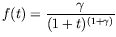(55)

where the packet loss situation corresponds to a condition that the packet will not leave one of the intermediate nodes in its route. Even when these works present interesting analytical results, there is no a comparison between these analytical results and the behavior observed in real data or in data obtained by computer simulations.

In  a theoretical model for network traffic is developed when the probability distribution function follows the expression given by equation (55). The equation of package migration can be expressed as a fractional derivative equation of the form: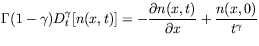(56)

If we assume the initial conditions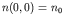and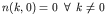we obtain: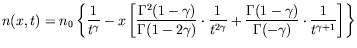(57)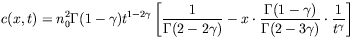(58)

where c(x,t) is the correlation function.

In our simulations a set of packages has to cross a number of intermediate nodes in order to go from the source to the destination. Different path lengths are possible. Each node maintains a queue of packages. One source node sends a file ofpackages to a destination node by a route of r intermediate step nodes. Each package has a time counter that represents the time that rests to send the package to the following node.

The following occurs at each time instant for each node.

1. The node sends all the packages that are ready (time counter equal 0) to the following node in the route.
2. The node decrements by one the time counter of all the packages remaining in its queue.
3. The node receives a set of packages from the previous node in the route and assigns a time delay to the package following a given probability distribution f(t).
At each time step t the number of packages n(x,t) at node x is exactly the size the node x queue.

The behavior of n(x,t) in our simulations is similar to the one predicted by theoretical model. We obtain a different behavior in the correlation c(x,t). The theoretical model predicts a growth in the value of c(x,t) as x or t increase and in our simulation a decrease in the value of c(x,t) is observed.

References
1
W.E. Leland , M.S. Taggu, Willinger and D.V. Wilson, "On the Self-Similar Nature of Ethernet Traffic". Proceedings of ACM SIGCOMM'93, San Francisco, 1993. doi:10.1145/167954.166255
2
S.M. Klivansky, A. Mukherjee and C. Song, "On Long-Range Dependence in NSFNET Traffic", Technical Report CIT-CC-94-61, 1994.
3
A. A. Kilbas, H. M. Srivastava and J. J. Trujillo, "Theory and Applications of Fractional Differential Equations", Elsevier, 2006.
4
V. Zaborovski, Y.Podgurski and S.Yegorov, New traffic model on the base of fractional calculus, Internet http://www.neva.ru/conf/art/art8.html

purchase the full-text of this paper (price £20)